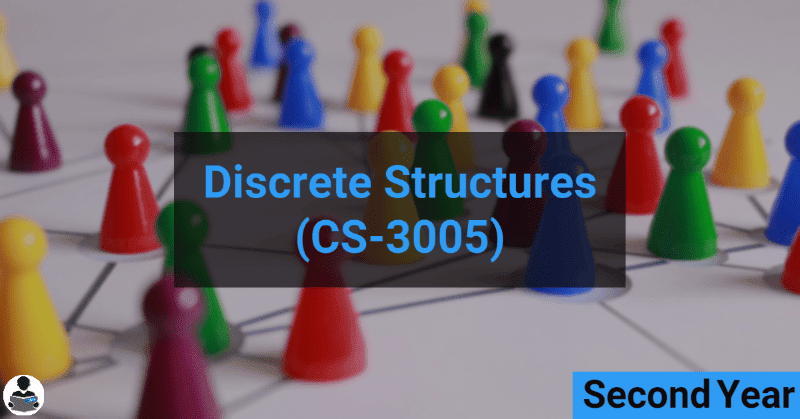Discrete Structures (CS-3005) - B.E RGPV CBCS & CBGS Scheme Notes -->
##### Discrete Structures (CS-3005)## Objective-

This course introduces the applications of discrete mathematics in the field of computer science. It covers sets, logic, proving techniques, combinatorics, functions, relations, Graph theory and algebraic structures. These basic concepts of sets, logic functions and graph theory are applied to Boolean Algebra and logic networks while the advanced concepts of functions and algebraic structures are applied to finite state machines and coding theory.

## Syllabus

UNIT 1:
Set Theory, Relation, Function, Theorem Proving Techniques : Set Theory: Definition of sets, countable and uncountable sets, Venn Diagrams, proofs of some general identities on sets Relation: Definition, types of relation, composition of relations, Pictorial representation of relation, Equivalence relation, Partial ordering relation, Job-Scheduling problem Function: Definition, type of functions, one to one, into and onto function, inverse function, composition of functions, recursively defined functions, pigeonhole principle. Theorem proving Techniques: Mathematical induction, Proof by contradiction.

UNIT 2:
Algebraic Structures: Definition, Properties, types: Semi Groups, Monoid, Groups, Abelian group, properties of groups, Subgroup, cyclic groups, Cosets, factor group, Permutation groups, Normal subgroup, Homomorphism and isomorphism of Groups, example and standard results, Rings and Fields: definition and standard results.

UNIT 3:
Propositional Logic: Proposition, First order logic, Basic logical operation, truth tables, tautologies, Contradictions, Algebra of Proposition, logical implications, logical equivalence, predicates, Normal Forms, Universal and existential quantifiers. Introduction to finite state machine Finite state machines as models of physical system equivalence machines, Finite state machines as language recognizers

UNIT 4:
Graph Theory: Introduction and basic terminology of graphs, Planer graphs, Multigraphs and weighted graphs, Isomorphic graphs, Paths, Cycles and connectivity, Shortest path in weighted graph, Introduction to Eulerian paths and circuits, Hamiltonian paths and circuits, Graph coloring, chromatic number, Isomorphism and Homomorphism of graphs.

UNIT 5:
Posets, Hasse Diagram and Lattices: Introduction, ordered set, Hasse diagram of partially, ordered set, isomorphic ordered set, well ordered set, properties of Lattices, bounded and complemented lattices. Combinatorics: Introduction, Permutation and combination, Binomial Theorem, Multimonial Coefficients Recurrence Relation and Generating Function: Introduction to Recurrence Relation and Recursive algorithms , Linear recurrence relations with constant coefficients, Homogeneous solutions, Particular solutions, Total solutions , Generating functions , Solution by method of generating functions.

• Unit 1
• Unit 2
• Unit 3
• Unit 4
• Unit 5

## Outcome:-

After this completion student will be familiar with relational algebra,Functions and graph theory.

## Books Recommended

1. C.L.Liu, “Elements of Discrete Mathematics” Tata Mc Graw-Hill Edition.
2. Trembley, J.P & Manohar; “Discrete Mathematical Structure with Application CS”, McGraw Hill.
3. Kenneth H. Rosen, “Discrete Mathematics and its applications”, McGraw Hill.
4. Bisht, “Discrete Mathematics”,Oxford University Press
5. Biswal,”Discrete Mathematics & Graph Theory”, PHI

## You May Also Like

#### Services

###### COMPLETELY FREE !!!

Yup, everything is free....

###### NO REGISTRATION REQUIRED

User doesn't have to register for accessing the files, all the files are free & universally accessible without any condition or restriction.

###### RESPONSIVE DESIGN & USER-FRIENDLY

Our webpages are responsive & user-friendly, which means it will automatically adjust according to your device screen size and you will find stuff without ant hustle.

All the files are uploaded on our super-fast servers so that they can be easily downloaded with high speed.

###### NEW PROJECTS

For providing a better experience to our users we are developing our Android application, the application will have a lot of awesome features so stay tuned ;).

###### AWESOME SUPPORT TEAM

Our AI-powered Chatbots are always here to help you so, feel free to ask any question or report if you face any problem. Our team also monitors all chatbots traffic & they will contact you if chatbot fails to help.